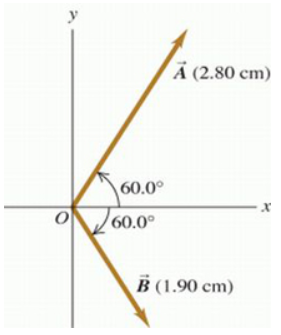# Problem: a) For the two vectors in the figure (Figure 1) , find the magnitude of the vector product A × B (cm2)b) Find the direction of the vector product A × B.options are (-z direction / +z direction)c) Find the magnitude of B × A (cm2)d) Find the direction of B × A.options are (-z direction / +z direction)

###### FREE Expert Solution

Cross product:

$\overline{)\stackrel{\mathbf{⇀}}{\mathbf{A}}{\mathbf{×}}\stackrel{\mathbf{⇀}}{\mathbf{B}}{\mathbf{=}}\stackrel{\mathbf{⇀}}{\mathbf{A}}\stackrel{\mathbf{⇀}}{\mathbf{B}}{\mathbf{s}}{\mathbf{i}}{\mathbf{n}}{\mathbf{\theta }}}$

91% (87 ratings)###### Problem Detailsa) For the two vectors in the figure (Figure 1) , find the magnitude of the vector product A × (cm2)

b) Find the direction of the vector product A × B.

options are (-z direction / +z direction)

c) Find the magnitude of B × A (cm2)

d) Find the direction of B × A.

options are (-z direction / +z direction)